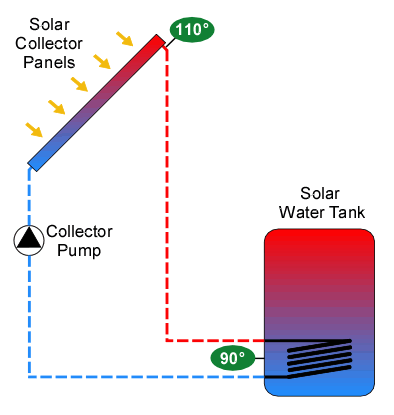# How Solar Thermal WorksA controller ( Steca TR 0603mc U) operates a solar thermal system by watching temperatures. In the typical system depicted here, only two temperatures are needed. These are the temperature of the water leaving the solar collectors, and the temperature of the water near the bottom of the solar water tank where the tank's heat exchanger is located. The Steca controller using factory settings will turn on the collector pump to ciculate the water when the difference between the collector temperature (Tc) and the tank temperature (Tt) exceeds 16° F. The pump will continue to run until that difference in temperature drops below 8° F. Using a different turn-on and turn-off temperature is called hysteresis. It is a technique used in all thermostats to keep equipment from constantly turning on and off as the temperature hovers about a single trip point. The turn-on temperature difference is fairly large so the pump can run for at least several minutes before depleting the heat stored in the collector. Cycling a pump on and off at a rapid rate increases the wear on the pump and uses more energy since starting a pump takes more power that keeping it running. When the pump runs, heated water is moved from the collectors to the heat exchanger in or surrounding the tank. On many browsers you can stop the animation by pressing the Esc key on your keyboard.Our thermal system uses two heat exchangers so we can heat both the water heater and the radiant floor. The controller works the same as described above, but now it monitors two sets of temperature differences to determine when to run the collector pump. The pump turns on if either temperature difference exceeds the threshold. At this point the controller operates the 3-way valve to choose which heat exchanger (tank or stand-alone) receives the heat. If the tank is receiving the heat, then everything proceeds at it would above in the examples above. When the stand-alone heat exchanger for the radiant floor is chosen, operation becomes more complicated. The controller manages the stand-alone heat exchanger with another pair of temperature sensors, exchanger temperature (Te) and floor temperature (Tf). As with the collector pump, it runs the exchanger pump when the temperature difference exceeds the programmed threshold. This threshold is set to a lower value than the collector pump due to the poor heat transfer into the concrete floor, ie the exchanger pump must run longer to do the same amount of heat transfer as occurs in metal pipes and tanks. Determining the amount of thermal power being produced is rather straight forward if one thinks in BTU (British Thermal Unit) instead of watts or joules. A BTU is approximately the amount of energy needed to increase the temperature of one pound of water (about a US pint) by one degree Fahrenheit. Power is BTUs per hour. Therefore by measuring the change in water temperature before and after the heat exchangers and multiplying by the flow rate (in pounds of water per hour) you get power in BTUs per hour. Multiplying BTU/hour by 3.412 converts to watts, or for energy: 3412 BTUs = 1 kWh. The flow rate is measured using a turbine flow rate sensor. With thermal power and pump power known, the system's coefficient of performance (COP) can be calculated. COP is the ratio of the amount of power generated over the amount of power used to create the generated power. Therefore COP is thermal power divided by pump power. An electric heater has a COP of 1. A good air conditioner would have a COP of around 4. This system runs with a COP between 15 and 20 in the winter and above 50 in the summer as shown in the Thermal COP Chart. On the animated graphic, flow rate is displayed below the collector pump in liters per minute (l/m) instead of the less familiar pounds per hour.